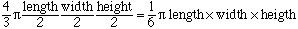SEARCH HOMEMath Central Quandaries & QueriesQuestion from dale: how many gallons in an oval shaped tank, 72in long x46.5in wide x23in deepDale,

If your oval is an ellipsoid then the volume is. Since your dimensions are in inches this expression will give you the volume in cubic inches. There are 231 cubic inches in a US gallon and 277.42 cubic inches in an Imperial gallon so divide the number of cubic inches by either 231 or 277.42 to obtain the number of gallons.

HarleyMath Central is supported by the University of Regina and The Pacific Institute for the Mathematical Sciences.